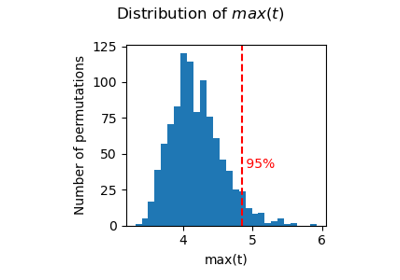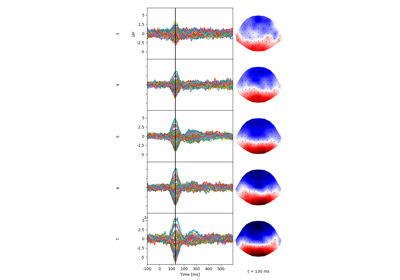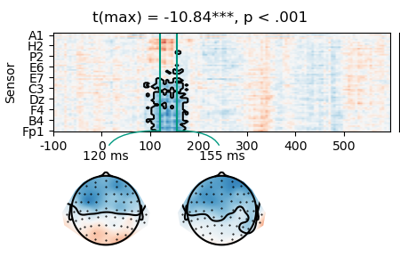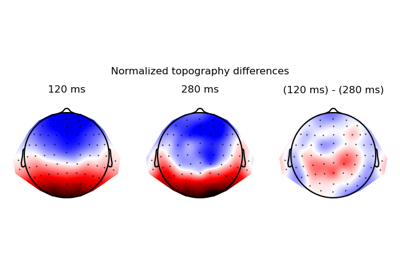# Mass-Univariate Statistics

With `NDVar` (“n-dimensional variables”), mass-univariate statistics can be evaluated analogous to univariate statistics.Permutation statistics

Permutation statisticsT-test

T-testMultiple regression

Multiple regressionTwo-stage test

Two-stage testCompare topographies

Compare topographies